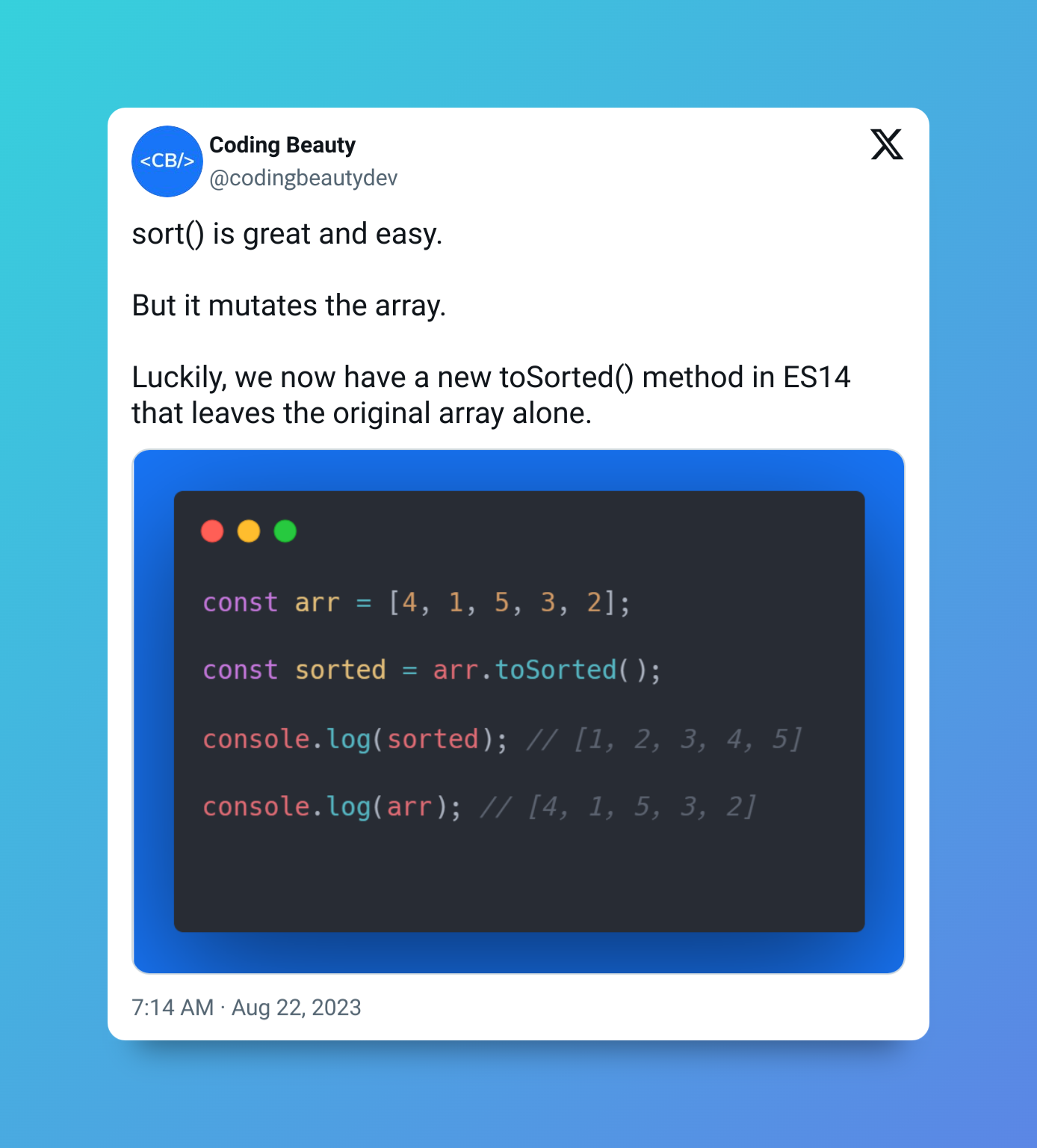# How to Convert Hours and Minutes to Seconds in JavaScript

To convert hours and minutes to seconds in JavaScript:

1. Multiply the hours by 3600 to convert it to seconds.
2. Multiply the minutes by 60 to convert it to seconds.
3. Add these seconds to the seconds time value to get the total seconds.

For example:

JavaScript
``````function toSeconds(hours, minutes, seconds) {
return hours * 3600 + minutes * 60 + seconds;
}

console.log(toSeconds(2, 15, 10)); // 8110

console.log(toSeconds(1, 5, 30)); // 3930
``````

We create a reusable `toSeconds()` function to easily convert the hours and minutes to seconds.

The function is quite easy to understand; 1 hour equals 3600 seconds, so we multiply the hour value by 3600 to get the seconds equivalent. Similarly, 1 minute equals 60 seconds, so we multiply the minute value by 60 to get the seconds equivalent.

After this, we add the equivalent seconds values to the `seconds` argument to get the total seconds.

We could rewrite the function to accept the time values as named properties of an object, instead of as multiple parameters.

JavaScript
``````function toSeconds({ h: hours, m: minutes, s: seconds }) {
return hours * 3600 + minutes * 60 + seconds;
}

console.log(toSeconds({ h: 2, m: 15, s: 10 })); // 8110

console.log(toSeconds({ h: 1, m: 5, s: 30 })); // 3930
``````

This approach makes it easier to understand the role of each value passed to the function, a## Convert `HH:mm:ss` to seconds in JavaScript

Sometimes the time input to convert to seconds is a string in a time format, like `HH:mm:ss`. To convert to seconds in this case, we’ll separate the individual time values by the separator (`:` in this case), cast them each to numbers, and perform the same time conversion steps done in our previous examples.

For example:

JavaScript
``````function toSeconds(timeStr) {
const [hours, minutes, seconds] = timeStr.split(':').map(Number);
return hours * 3600 + minutes * 60 + seconds;
}

console.log(toSeconds('02:15:10')); // 8110

console.log(toSeconds('01:05:30')); // 3930
``````

The input string here is in the `HH:mm:ss` format; the hour, minute, and second values are separated by a colon (`:`) and are each represented by a minimum of 2 digits.

The `String` `split()` method splits a string into an array of substrings separated by a given separator in the original string. We pass a colon as the separator to get an array of the individual time values.

JavaScript
``````const timeStr = '02:15:10';

console.log(timeStr.split(':')); // [ '02', '15', '10' ]
``````

After getting this array, we use the `map()` method to transform each time value into a number. The `map()` method takes a callback and calls it on each element of an array and uses the result to populate a new array. For our scenario, the callback is simply the `Number()` constructor, so each time value in the array is converted to a number.

JavaScript
``console.log(['02', '15', '10'].map(Number)); // [ 2, 15, 10 ]``

Note

This:

JavaScript
``const [hours, minutes, seconds] = timeStr.split(':').map(Number);``

is equivalent to this:

JavaScript
``````  const [hours, minutes, seconds] = timeStr
.split(':')
.map((str) => Number(str));``````

The second one is longer, but it makes it clear exactly what arguments are passed to the `map()` callback. In the first one, `map()` automatically passes 3 arguments to its callback, which could be problematic if the callback returns a different result depending on the number of arguments it receives, for instance, a `parseInt()` callback.

We then use a destructuring assignment to unpack the number array values into separate hour, minute, and second variables.

JavaScript
``````const [hour, minute, seconds] = [2, 15, 10];

console.log(hour); // 2

console.log(minute); // 15

console.log(seconds); // 10
``````

After doing this, we perform the same multiplication and addition we did in the first example, to convert the hours and minutes to seconds and get the total seconds.